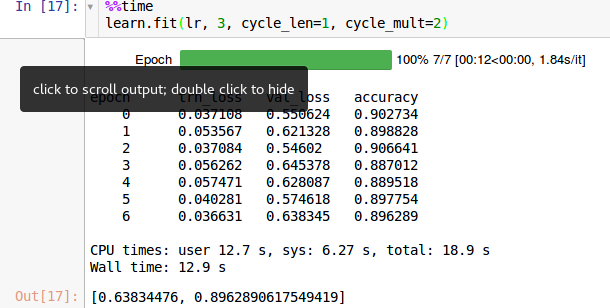# Running learn.fit multiple times

I noticed when I run learn.fit multiple times (over again), trn_loss is getting smaller and smaller after each time.Is there a way to ‘clean’ earlier iteration from memory, so that it don’t occur? (for now I’m restarting kernel :/)
here is screenshot how it looks ‘fresh’ after restatring kernel:

Rerun the "learn = " step and any other steps that use that learn variable.

2 Likes

Thanks! It worked perfectly

I have a similar question. I do notice the same when I train the last layer:

learn.precompute = False
learn.fit(1e-2, 3, cycle_len = 1)

Or, the multiple layers:

learn.unfreeze()
lr=np.array([1e-4,1e-3,1e-2])
learn.fit(lr, 4, cycle_len=1, cycle_mult=2)

-My question is, is this wrong? Does it overfit the model?
-What input arguments in `learn.fit` should I tweak to achieve the best training without overfitting?